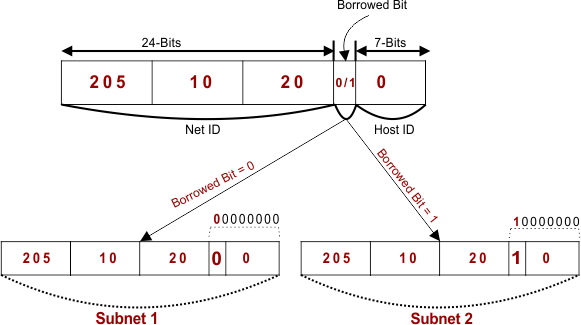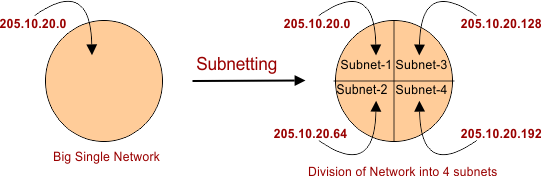Select Page

# Subnetting In ClaasFul IP Addressing

As we already know that, The classful subnetting is also called Fixed length subnetting. The Fixed length subnetting contain  the following properties.

• Sizes of all sub networks are same.
• Subnets of all sub networks are same.
• All the sub networks have equal number of hosts.

Let understand with example

## Example-01

Suppose

• We have a big single big network having IP Address 205.10.20.0.
• We want subnetting. So, divide this network into 2 subnets.

As given IP belongs to Class C So, 24 bits are used for net ID and 8 bits are used for Host ID.For creating two subnets (sub networks) and to represent their subnet IDs, we require 1 borrowed-bit from Host part.

So,

• We borrowed one bit from the Host part.
• If borrowed bit = 0, then it will represent the first subnet.
• If borrowed bit = 1, then it will represent the second subnet.Note: After borrowing one bit, Host ID part remains with only 7 bits.

IP Address of the two subnets are

• 10.20.00000000 = 205.10.20.0
• 10.20.10000000 = 205.10.20.128

## For First Subnet

• IP Address of the subnet = 205.10.20.0
• Total number of IP Addresses = 27= 128
• Total number of hosts = 128 – 2 = 126
• Range of IP Addresses = [205.10.20. 00000000 to 205.10.20. 01111111] = [205.10.20.0 to 205.10.20.127]

## For Second Subnet

• IP Address of the subnet = 205.10.20.128
• Total number of IP Addresses = 27= 128
• Total number of hosts = 128 – 2 = 126
• Range of IP Addresses = [205.10.20.10000000 to 205.10.20.11111111] = [205.10.20.128 to 205.10.20.255]

Important Note: Number of subnets depends upon the number of borrowed bits. We can define this relation through the following formula.

Number of subnets = 2n. where “n” is number of borrowed bits. So, if number of borrowed bits are 2 then subnets will be 22 = 4.

If given IP= 205.10.20.0. then first IP Address of each of four subnets are

• 10.20.00000000 = 200.1.2.0
• 10.20.01000000 = 200.1.2.64
• 10.20.10000000 = 200.1.2.128
• 10.20.11000000 = 200.1.2.192Help Other’s By Sharing…